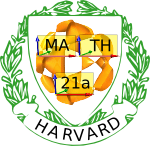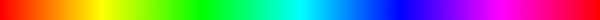Fall 2005

# Mathematics Math21a Fall 2005

## Multivariable Calculus

Office: SciCtr 434
Email: knill@math.harvard.edu
Harvard Mathematics

 Weekly checklists: "Somewhere, over the rainbow ..."Week1 Week2 Week3 Week4 Week5 Week6 Week7 Week8 Week9 Week10 Week9 Week10

## Mathematica Laboratory

Deadline The Mathematica project is due the last day of class. That means Monday December 19'th for the Mo/We/Fr sections, and Tuesday December 20'th for the Tue/Thu sections. You hand in the Mathematica project printout with the last homework. We need a physical printout which contains the graphics and answers to the questions at the end of the notebook. Show your work. Also for the graphics plots, we need to see the command, which produced the plot.
Availability Mathematica is installed on some computers in the basement of the Science center. It might also be installed on some computers in the houses. If you want to try an installation of Mathematica on your own computer, get it here. Note that you have to be on a Harvard network and have your PIN ready to download the software and that requesting the Mathematica Password requires you to send the request from a Harvard computer.
Installation After you downloaded the program to your computer, start the application and follow the instructions. During the installation progress, you have to enter the Harvard Licence number L2482-2405. The number which you will get in return has to be entered in the Mathematica Registration page. You will then be sent a password by email. This is what you see during installation in Send email to math21a@fas, if you plan to use Mathematica on a linux system.
Getting the notebook
• The Mathematica laboratory assignment is

## here

The actual assignments are at the end of the notebook.
Running mathematica Mathematica is started like any other application on Macintoshs or PC's. On Linux, just type "mathematica" to start the notebook version, or "math" to start the terminal version.
Some frequently used commands: ( See more what you can do with Mathematica)
 Example how to check homework: change the function and get an analysis about its critical points: ``` (* Mathematica code to classify critical points, O. knill, 2000 *) f[x_,y_]:=4 x y - x^3 y - x y^3; a[x_,y_]:=D[f[u,v],u] /. {u->x,v->y}; b[x_,y_]:=D[f[u,v],v] /. {u->x,v->y}; A=Solve[{a[x,y]==0,b[x,y]==0},{x,y}]; CriticalPoints=Table[{A[[i,1,2]],A[[i,2,2]]},{i,Length[A]}]; H[{x_,y_}]:={{D[f[u,v],{u,2}],D[D[f[u,v],v],u]},{D[D[f[u,v],u],v],D[f[u,v],{v,2}]}} /. {u->x,v->y}; F[A_]:=A[[1,1]]; Discriminant=Map[Det,Map[H,CriticalPoints]] FirstEntry=Map[F,Map[H,CriticalPoints]] Decide[B_]:=If[Det[B]<0,"saddle",If[B[[1,1]]<0,"max","min"]]; Analysis=Map[Decide, Map[H,CriticalPoints]]; Table[{CriticalPoints[[i]],Analysis[[i]]},{i,Length[CriticalPoints]}] ```

 Plot[ x Sin[x],{x,-10,10}] Graph function of one variable Plot3D[ Sin[x y],{x,-2,2},{y,-2,2}] Graph function of two variables ParametricPlot[ {Cos[3 t],Sin[5 t]} ,{t,0,2Pi}] Plot planar curve ParametricPlot3D[ {Cos[t],Sin[t],t} ,{t,0,4Pi},AspectRatio->1] Plot space curve ParametricPlot3D[ {Cos[t] Sin[s],Sin[t] Sin[s],Cos[s]},{t,0,2Pi},{s,0,Pi}] Parametric Surface ContourPlot[ Sin[x y],{x,-2,2},{y,-2,2} ] Contour lines (traces) Integrate[ x Sin[x], x] Integrate symbolically NIntegrate[ Exp[-x^2],{x,0,10}] Integrate numerically D[ Cos^5[x],x ] Differentiate symbolically Series[Exp[x],{x,0,3} ] Taylor series DSolve[ x''[t]==-x[t],x,t ] Solution to ODE Get["Graphics`ContourPlot3D`"]; ContourPlot3D[x^2+2y^2-z^2-1,{x,-2,2},{y,-2,2},{z,-2,2}] Implicit surface
Printing problems
• I tried to print my notebook but there is no printout.
1. Check your printing budget. The printer would spit out a page, which tells you this.
2. You might have written the notebook to a PS file on your computer instead of sending it to the printer (you can chose so at the printing dialog box).
• I want to save paper. How do I print out only a part of the notebook.
1. Chose "print selection" instead of "print" in the Mathematica notebook. The selected cells will be printed.
2. Alternatively, open a new notebook, cut and paste the part you need to turn in into the new notebook and print that out.

 Please send comments to math21a@fas.harvard.edu Background music: Pictures at an Exhibition" by the Russian composer Modest Petrovich Mussorgsky.
 Math21a, Multivariable Calculus, Fall 2005, Department of Mathematics, Faculty of Art and Sciences, Harvard University

Sat Jan 21 22:11:28 EST 2006